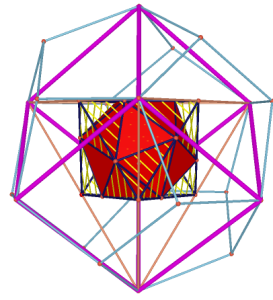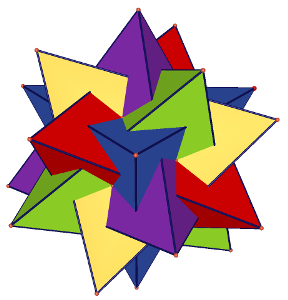Duality Property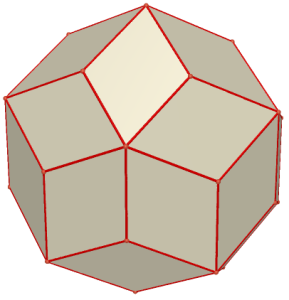biggest dodecahedron in icosahedron

diamond 30 faces polyhedron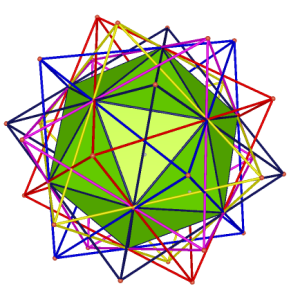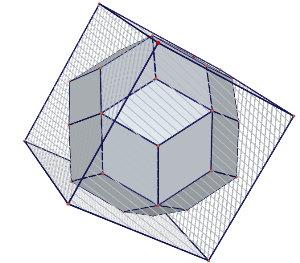icosahedron in 5 octahedrons

make a cube from diamond 30 faces polyhedronmake a cube from icosahedron

make a cube from octahedron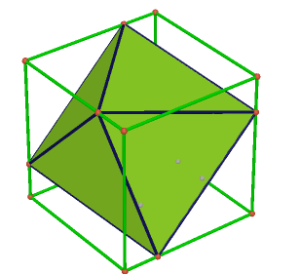make a icosahedron from octahedron

make a octahedron from cube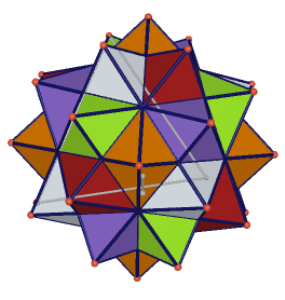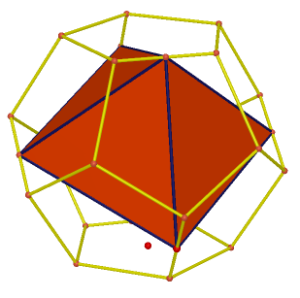Octahedron 5-Compound

octahedron in dodecahedron